Author: Oscar Cronquist Article last updated on July 01, 2022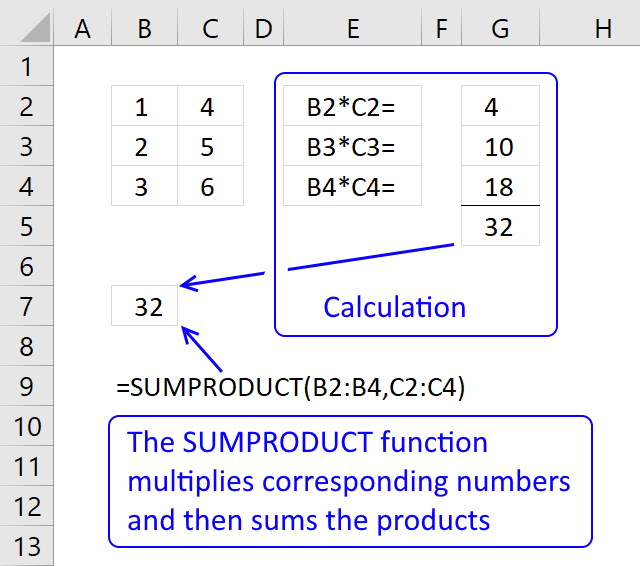The SUMPRODUCT function calculates the product of corresponding values and then returns the sum of each multiplication.

The picture above shows how the SUMPRODUCT works in greater detail.

Formula in cell B7:

=SUMPRODUCT(B2:B4, C2:C4)

The SUMPRODUCT function multiplies cell ranges row by row and then adds the numbers and returns a total. The formula in cell B7 multiples values 1*4= 4, 2*5 = 10 and 3*6 =18 and then adds the numbers 4+10+18 equals 32.

## 1. Excel Function Syntax

SUMPRODUCT(array1, [array2], ...)

## 2. Arguments

 array1 Required. Required. The first array argument whose numbers you want to multiply and then sum. [array2] Optional. Up to 254 additional arguments.

## 3. Things to know about the SUMPRODUCT function

The SUMPRODUCT function is one of the most powerful functions in Excel and is one that I often use. I highly recommend learning how it works.

The SUMPRODUCT function requires you to enter it as a regular formula, not an array formula. However, there are exceptions. If you use a logical expression you must enter the formula as an array formula and convert the boolean values to their equivalents.

There are workarounds to this problem which I will demonstrate in the examples below.

Note, Excel 365 subscribers do not need to enter formulas as array formulas in the examples below. Microsoft has changed how array formulas work and they are now called "dynamic arrays".

## 4. How to use the SUMPRODUCT function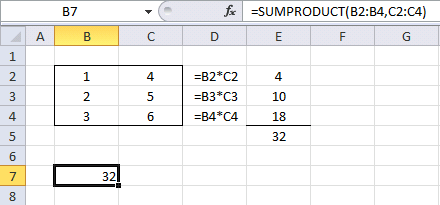This example demonstrates how the SUMPRODUCT function works.

Formula in cell B7:

=SUMPRODUCT(B2:B4, C2:C4)

### 4.1 Explaining formula

#### Step 1  - Multiplying values on the same row

The first array is in cell range B2:B4 and the second array is in cell range C2:C4.

B2:B4*C2:C4

becomes

{1;2;3} * {4;5;6}

becomes

{1*4; 2*5; 3*6}

and returns {4; 10; 18}.

The same calculations are done in column D and shown in column E, see above picture.

#### Step 2 - Return the sum of those products

{4; 10; 18}

becomes

4 + 10 + 18

and the function returns 32 in cell B7.

The same calculation is done E5, the sum of the products in cell range E2:E4 is calculated in cell E5. See the above picture.

Now you know the basics. Let's move on to something more interesting.

## 5. How to use a logical expression in the SUMPRODUCT functionThe image above demonstrates a formula in cell G4 that counts how many cells that is equal to the value in cell G2. Note, that this is only an example. I recommend you use the COUNTIF function to count cells based on a condition, it is designed to do that.

Formula in cell G4:

=SUMPRODUCT(--(B2:B6=\$G\$2))

### 5.1 Explaining formula

#### Step 1  - Logical expression returns a boolean value that we must convert to numbers

There is only one array in this formula but something else is distorting the picture. A comparison operator (equal sign) and a second cell value (G2) or a comparison value. With these, we have now built a logical expression. This means that the value in cell G2 is compared to all the values in cell range B2:B6 (not case sensitive).

B2:B6=\$G\$2

becomes

and returns

{FALSE; TRUE; FALSE; TRUE; FALSE}.

They are all boolean values and excel can´t sum these values. We have to convert the values to numerical values. There are a few options, you can:

• Add a zero - (B2:B6=\$G\$2)+0
• Multiply with 1 - (B2:B6=\$G\$2)*1
• Double negative signs  --(B2:B6=\$G\$2)

They all convert boolean values to their numerical equivalents. TRUE is 1 and FALSE is 0 (zero).

--( {FALSE;TRUE;FALSE;TRUE;FALSE})

becomes

{0;1;0;1;0}

#### Step 2 - Return the sum of those products

{0;1;0;1;0}

becomes

0 + 1 + 0 + 1 + 0

and returns 2 in cell G4. There are two cells containing the value "California" in cell range B2:B6.

You accomplish the same thing using the COUNTIF function or count multiple values in different columns using the COUNTIFS function. In fact, you can count entire records in a data set using the COUNTIFS function.

## 6. How to use multiple conditions in the SUMPRODUCT function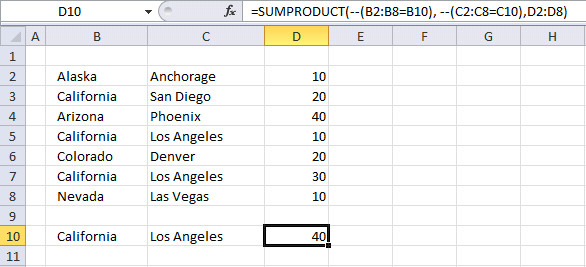This example shows how to use multiple conditions in the SUMPRODUCT function using AND logic. AND logic means that all conditions must be met on a given row in order to add the number to the total.

Formula in cell D10:

=SUMPRODUCT(--(B2:B8=B10), --(C2:C8=C10),D2:D8)

This formula contains three arguments, the SUMPRODUCT function allows you to use up to 30 arguments. You can make the formula somewhat shorter:

=SUMPRODUCT((B2:B8=B10)*(C2:C8=C10)*D2:D8)

This also allows you to have a lot more conditions than 30 if you like and use OR logic if you want. Example 4 demonstrates OR logic.

It is only the available computer memory that is the limit if you use this method. The formula looks like an array formula but no, you are not required to enter it as an array formula.

### 6.1 Explaining formula

#### Step 1  - Multiplying corresponding components in the given arrays

The first logical expression B2:B8=B10 uses an equal sign to check if the values in cell range B2:B8 are equal to the value in cell B10.

B2:B8=B10

becomes

and returns

{FALSE; TRUE; FALSE; TRUE; FALSE; TRUE; FALSE}.

The second logical expression is C2:C8=C10, the other logical operators you can use are:

• = equal
• < less than
• > greater than
• <> not equal to
• <= less than or equal to
• >= larger than or equal to

C2:C8=C10

becomes

{"Anchorage";"San Diego";"Phoenix";"Los Angeles";"Denver";"Los Angeles";"Las Vegas"}="Los Angeles"

and returns

{FALSE; FALSE; FALSE; TRUE; FALSE; TRUE; FALSE}.

The third and last logical

(B2:B8=B10)*(C2:C8=C10)*D2:D8

becomes

({FALSE; TRUE; FALSE; TRUE; FALSE; TRUE; FALSE})*({FALSE; FALSE; FALSE; TRUE; FALSE; TRUE; FALSE})*{10; 20; 40; 10; 20; 30; 10}

becomes

{0;0;0;1;0;1;0}*{10; 20; 40; 10; 20; 30; 10}

and returns

{0; 0; 0; 10; 0; 30; 0}

#### Step 2 - Return the sum of those products

{0; 0; 0; 10; 0; 30; 0}

becomes

10 +30

and returns 40 in cell D10.

## 7. How to use OR logic in the SUMPRODUCT function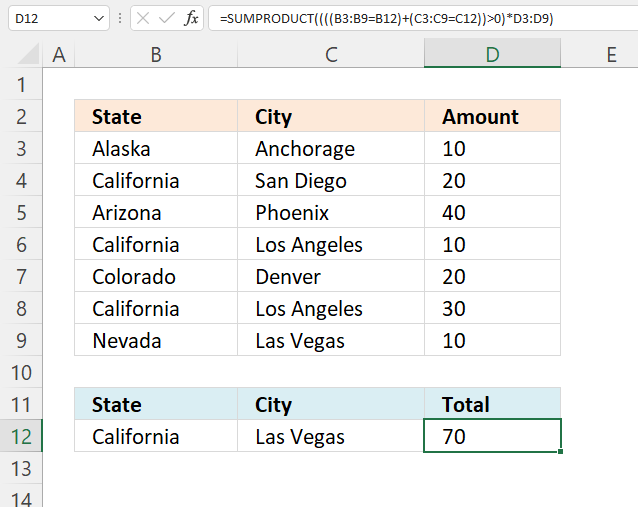This example shows how to use multiple conditions. A number in cell range D3:D9 is added if the item in cell B12 is found on the corresponding row in cell range B3:B9 OR if the item in C12 is found in cell range C3:C9.

Formula in cell D10:

=SUMPRODUCT(((B3:B9=B12)+(C3:C8=C12))*D3:D9)

### Explaining formula in cell D10

#### Step 1 - Compare values to condition

The equal sign lets you compare the condition to values in B3:B9, the result is an array with the same size as B3:B9 containing TRUE or FALSE.

B3:B9=B12

becomes

and returns

{FALSE; TRUE; FALSE; TRUE; FALSE; TRUE; FALSE}.

#### Step 2 - Compare values to condition

C3:C8=C12

becomes

{"Anchorage"; "San Diego"; "Phoenix"; "Los Angeles"; "Denver"; "Los Angeles"; "Las Vegas"}="Las Vegas"

and returns

{FALSE; FALSE; FALSE; FALSE; FALSE; FALSE; TRUE}.

#### Step 3 - Apply OR logic

We need to add numbers if at least one of the conditions matches on the same row, this means OR logic.

Mathematical operators between arrays allow you to do more complicated calculations, like this:

* (asterisk) - Both logical expressions must match (AND logic)

+ (plus sign) - Any of the logical expressions must match, it can be all it can be only one. It doesn't matter. (OR logic)

The AND logic behind this is that

• TRUE * TRUE = TRUE (1)
• TRUE * FALSE = FALSE (0)
• FALSE * FALSE = FALSE (0)

The OR logic works like this:

• TRUE + TRUE = TRUE (1)
• TRUE + FALSE = TRUE (1)
• FALSE + FALSE = FALSE (0)

The numbers above show the numerical equivalent of each result. True equals 1 and False equals 0 (zero).

The parentheses shown below let you control the order of calculation, we must do the comparisons before we add the arrays.

(B2:B8=B10)+(C2:C8=C10)

becomes

{FALSE; TRUE; FALSE; TRUE; FALSE; TRUE; FALSE} + {FALSE; FALSE; FALSE; FALSE; FALSE; FALSE; TRUE}

and returns {0; 1; 0; 1; 0; 1; 1}.

#### Step 4 - Check if result is larger than 0 (zero)

If both values match the conditions the result is two, the result will be twice as much. We can't multiply with two, the larger than character checks if the result is larger than 0 (zero).

((B3:B9=B12)+(C3:C9=C12))>0

becomes

{0; 1; 0; 1; 0; 1; 1}>0

and returns {FALSE; TRUE; FALSE; TRUE; FALSE; TRUE; TRUE}.

#### Step 5 - Multiply with numbers

((B2:B8=B10)+(C2:C8=C10))*D2:D8

becomes

{FALSE; TRUE; FALSE; TRUE; FALSE; TRUE; TRUE}*D2:D8

becomes

{FALSE; TRUE; FALSE; TRUE; FALSE; TRUE; TRUE}*{10; 20; 40; 10; 20; 30; 10}

and returns {0; 20; 0; 10; 0; 30; 10}.

SUMPRODUCT(((B2:B8=B10)+(C2:C8=C10))*D2:D8)

becomes

SUMPRODUCT({0; 20; 0; 10; 0; 30; 10})

and returns 70.

These expressions check if California is found in cell range B2:B8 or Las Vegas is found in cell range C2:C8. They are found in rows 4, 6,8, and 9.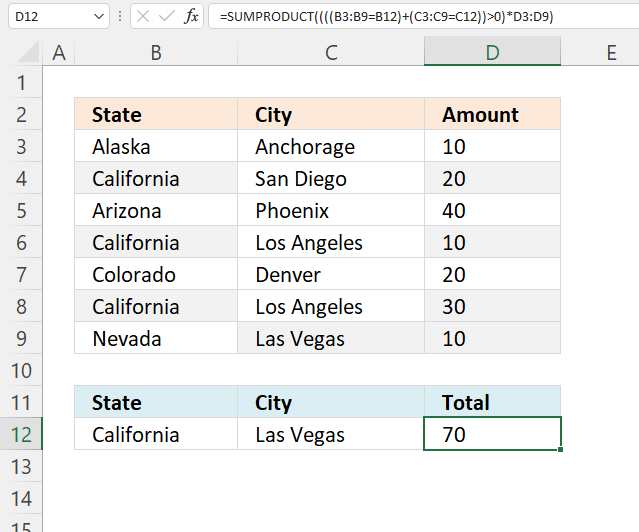The SUMPRODUCT function sums the corresponding values in column D and returns 70 in cell D10. 20+10+30+10 equals 70.

### Get the Excel fileSumproduct-function.xlsx